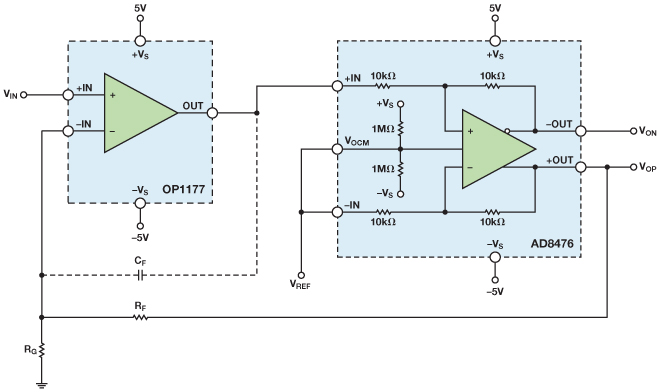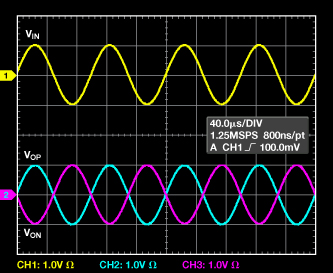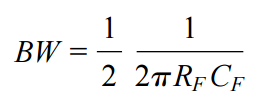# Versatile, Low-Power, Precision Single-Ended-to-Differential Converter

Many applications, including driving modern analog-to-digital converters (ADCs), transmitting signals over twisted-pair cables, and conditioning high-fidelity audio signals, require differential signaling, which achieves higher signal-to-noise ratios due to the use of larger signals for a given set of power supply voltages, increased common-mode noise immunity, and lower second-harmonic distortion. This requirement presents a need for a circuit block that can convert single-ended signals, found in most signal chains, to differential signals.

Figure 1 shows a simple single-ended-to-differential converter using an AD8476 precision, low power, fully differential amplifier (diff-amp) with integrated precision resistors. The diff-amp is internally configured for a differential gain of one, so the circuit’s transfer function is

 VOUT, DIFF = VOP – VON = VIN.

The output common-mode voltage, (VOP + VON)/2, is set by the voltage on the VOCM pin. If the VOCM pin is allowed to float, the output common-mode voltage will float to midsupply due to internal 1-MΩ resistors that form a resistance divider with the power supplies. Capacitor C1 filters the noise of the 1-MΩ resistors to lower the output common-mode noise. The circuit’s gain error is only 0.04% maximum due to the AD8476’s internal laser-trimmed gain-setting resistors.Figure 1. Simple single-ended-to-differential converter.

For many applications, the circuit in Figure 1 is more than adequate to perform the single-ended-to-differential conversion. For applications that require improved performance, Figure 2 shows a single-ended-to-differential converter that has very high input impedance, 2-nA maximum input bias current, 60-µV maximum offset (RTI), and 0.7-µV/°C maximum offset drift. The circuit achieves this level of performance by cascading an OP1177 precision operational amplifier (op amp) with the AD8476 and feeding back the positive output voltage of the AD8476 to the inverting input of the op amp. This feedback arrangement allows the op amp to determine the precision and noise performance of the configuration since it connects the diff-amp inside a feedback loop with an op amp’s large open-loop gain preceding it. As a result, the errors of the AD8476—including noise, distortion, offset, and offset drift—are reduced by this large gain when referred to the input.Figure 2. Improved single-ended-to-differential converter.

The circuit in Figure 2 is described by the following equations:

 VOP ≈ VIN (1)
 (VOP + VON) = 2 VOCM = 2 VREF (2)
 VON = 2 VREF – VIN (3)

Combining (1) and (3),

 VOUT, DIFF = VOP – VON = 2(VIN – VREF) (4)

Equation 3 demonstrates two important properties about the circuit: first, the circuit’s single-ended-to-differential gain is two. Second, the VREF node serves as the reference for the input signal, so it can be used to null out an offset in the input signal. For example, if the input signal has a 1-V offset, applying 1-V to the VREF node nulls it out.

If the intended application requires a gain greater than two, the circuit in Figure 2 can be modified, as shown in Figure 3. In this case, the single-ended-to-differential gain of the circuit is dictated by external resistors, RF and RG, such that:(5)

and(6)Figure 3. Improved single-ended-to-differential converter with resistor-programmable gain.

Like the circuit in Figure 2, this improved single-ended-to-differential converter rejects the errors of the diff-amp by placing it inside the op amp’s feedback loop. As with any feedback connection, care must be taken to make sure the system is stable. Referring to Figure 2, the cascade of the OP1177 and the AD8476 form a composite differential-out op amp where the open-loop gain over frequency is the product of the op amp’s open-loop gain and the diff-amp’s closed-loop gain. Therefore, the closed-loop bandwidth of the AD8476 adds a pole to the open-loop gain of the OP1177. To ensure stability, the bandwidth of the diff-amp should be higher than the unity-gain frequency of the op amp. This requirement is relaxed in the circuit of Figure 3, as the resistive feedback network effectively reduces the unity-gain frequency of the OP1177 by a factor of RG/(RG + RF). Since the AD8476 has a bandwidth of 5 MHz and the OP1177 has a unity-gain frequency of 1 MHz, the circuits shown do not exhibit stability issues. Figure 4 shows an oscilloscope photo of the input and output signals of the circuit in Figure 2 when driven with a ground-referenced 10-Hz, 1-V p-p sinusoid. For simplicity, the VREF node was tied to ground.Figure 4. Input and output signals of the circuit in Figure 2 when driven with a ground-referenced 10-kHz, 1-V p-p sinusoid.

When using an op amp where the unity gain frequency is much larger than the diff-amp’s bandwidth, a bandwidth-limiting capacitor, CF, can be inserted, as shown in Figure 3. Capacitor CF forms an integrator with the feedback resistor, RF, such that the bandwidth of the overall circuit is given by(7)

The ½ in the bandwidth equation is due to the feedback being single-ended rather than differential, which reduces the feedback and bandwidth by a factor of 2. If this reduced bandwidth is lower than the closed-loop bandwidth of the diff-amp, the circuit will be stable. This bandwidth-limiting technique can also be employed with a gain of two by making RG an open circuit.